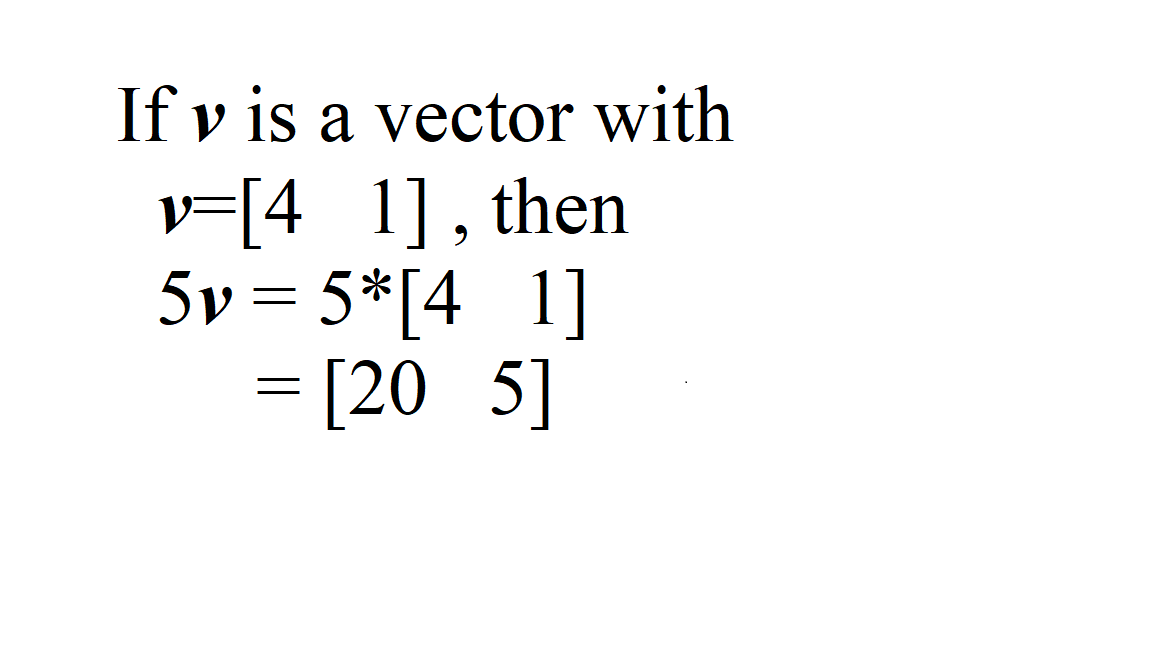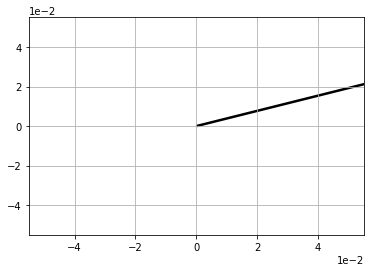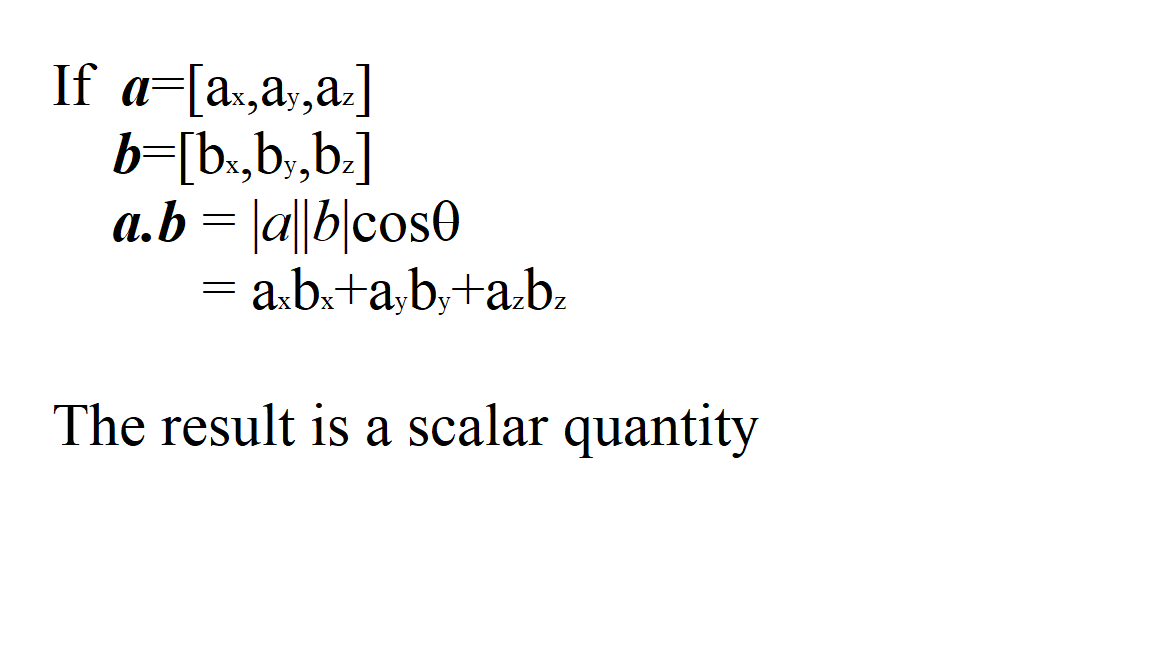# NumPy | Vector Multiplication

Vector multiplication is of three types:

• Scalar Product
• Dot Product
• Cross Product
• Scalar Multiplication:
Scalar multiplication can be represented by multiplying a scalar quantity by all the elements in the vector matrix.Code: Python code explaining Scalar Multiplication

 `      `  `# importing libraries   ` `import` `numpy as np ` `import` `matplotlib.pyplot as plt ` `import` `math ` ` `  `v ``=` `np.array([``4``, ``1``]) ` `w ``=` `5` `*` `v ` `print``(``"w = "``, w) ` ` `  `# Plot w ` `origin ``=``[``0``], [``0``] ` `plt.grid() ` `plt.ticklabel_format(style ``=``'sci'``, axis ``=``'both'``,  ` `                     ``scilimits ``=``(``0``, ``0``)) ` `plt.quiver(``*``origin, ``*``w, scale ``=` `10``) ` `plt.show() `

Output :

`w =  [20  5]`Dot Product multiplication:Code: Python code to explain Dot Product Multiplication

 `import` `numpy as np ` `import` `math ` ` `  `v ``=` `np.array([``2``, ``1``]) ` `s ``=` `np.array([``3``, ``-``2``]) ` `d ``=` `np.dot(v, s) ` `print``(d) `

Here, dot product can also be received using the ‘@’ operator.

d = v@s

Output :

`4`

Code: Python code explaining Cross Product

 `import` `numpy as np ` `import` `math ` ` `  `v ``=` `np.array([``4``, ``9``, ``12``]) ` `s ``=` `np.array([``21``, ``32``, ``44``]) ` `r ``=` `np.cross(v, s) ` `print``(r) `

Output:

`[ 12  76 -61]`

Attention geek! Strengthen your foundations with the Python Programming Foundation Course and learn the basics.

To begin with, your interview preparations Enhance your Data Structures concepts with the Python DS Course.

My Personal Notes arrow_drop_upCheck out this Author's contributed articles.

If you like GeeksforGeeks and would like to contribute, you can also write an article using contribute.geeksforgeeks.org or mail your article to contribute@geeksforgeeks.org. See your article appearing on the GeeksforGeeks main page and help other Geeks.

Please Improve this article if you find anything incorrect by clicking on the "Improve Article" button below.

Article Tags :

2

Please write to us at contribute@geeksforgeeks.org to report any issue with the above content.